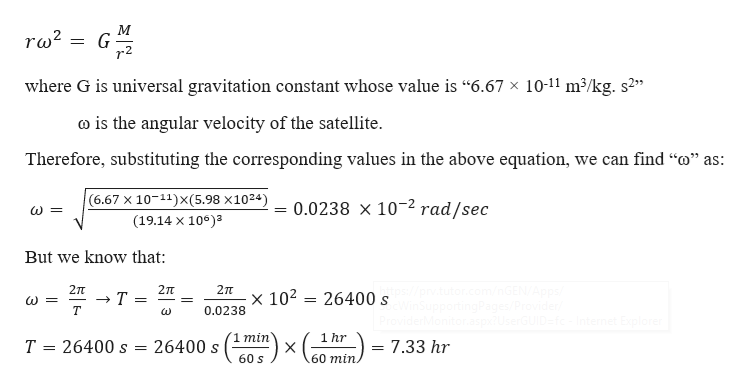A satellite circles the earth in an orbit whose radius is 2.20 times the earth's radius. The earth's mass is 5.98 x 1024 kg, and its radius is 6.38 x 106 m. What is the period of the satellite?

Question

A satellite circles the earth in an orbit whose radius is 2.20 times the earth's radius. The earth's mass is 5.98 x 1024 kg, and its radius is 6.38 x 106 m. What is the period of the satellite?

Step 1

Given information:

Radius of earth (R) = 6.38 × 106 m

Radius of the satellite orbit (r) = 3R = 19.14 × 106 m

Mass of the earth (M) = 5.98 × 1024 kg

Step 2

From Newton’s law of gravitation, the centripetal force acting on the satell...help_outlineImage TranscriptioncloseМ ra2 where G is universal gravitation constant whose value is "6.67 x 10-11 m3/kg. s2 co is the angular velocity of the satellite Therefore, substituting the corresponding values in the above equation, we can find "o" as: (6.67 X 10 11)X(5.98 x1024) 0.0238 x 10 2 rad/sec (19.14 x 10)3 But we know that com inGEN/Aops / Provider 2T 2л 2TT x 102 26400 s Т т 0.0238 Internet Explorer 1 min 1 hr 26400 s 26400 s Т 7.33 hr X 60 min. 60 s fullscreen

Want to see the full answer?

See Solution

Want to see this answer and more?

Our solutions are written by experts, many with advanced degrees, and available 24/7

See Solution
Tagged in

Angular Motion